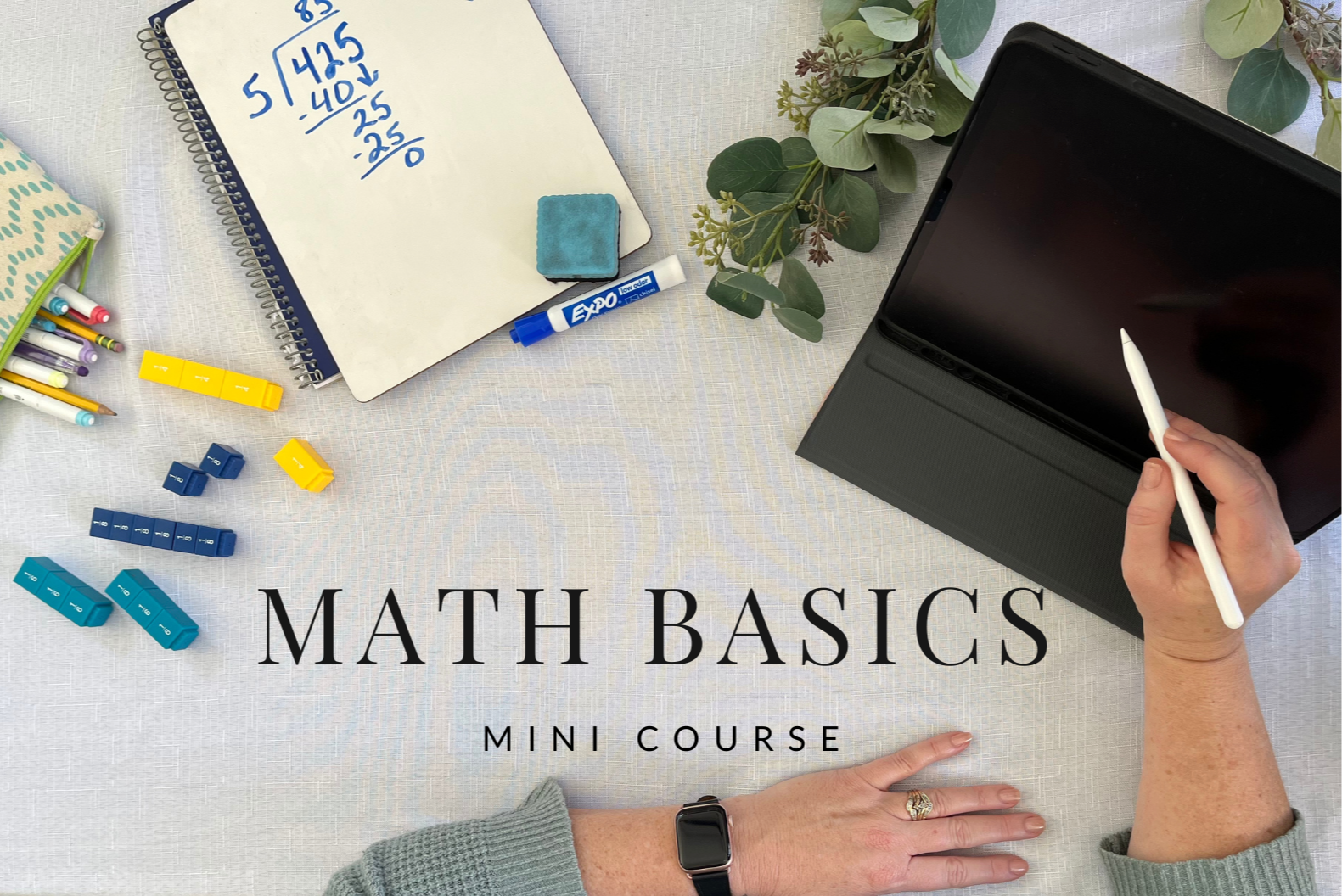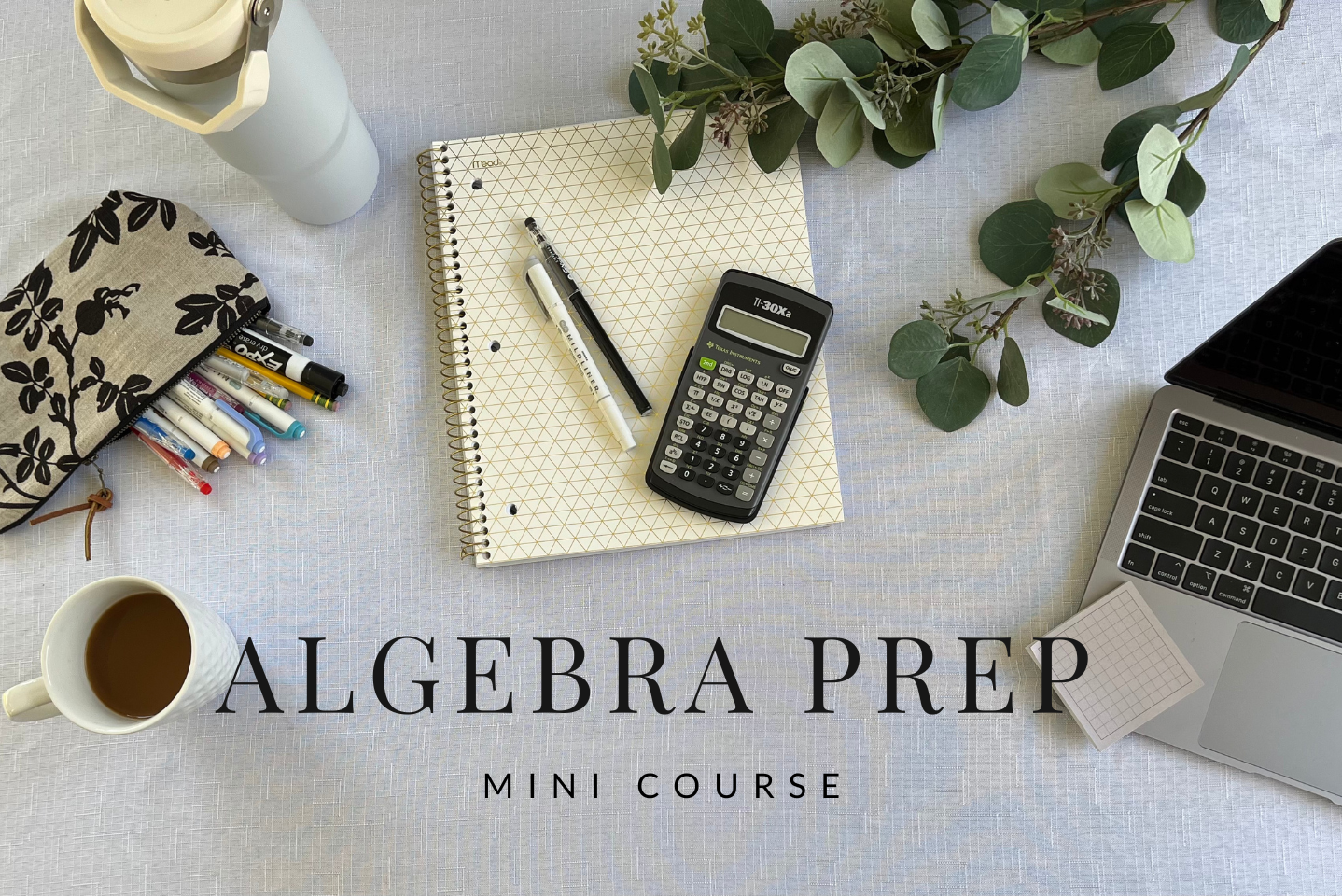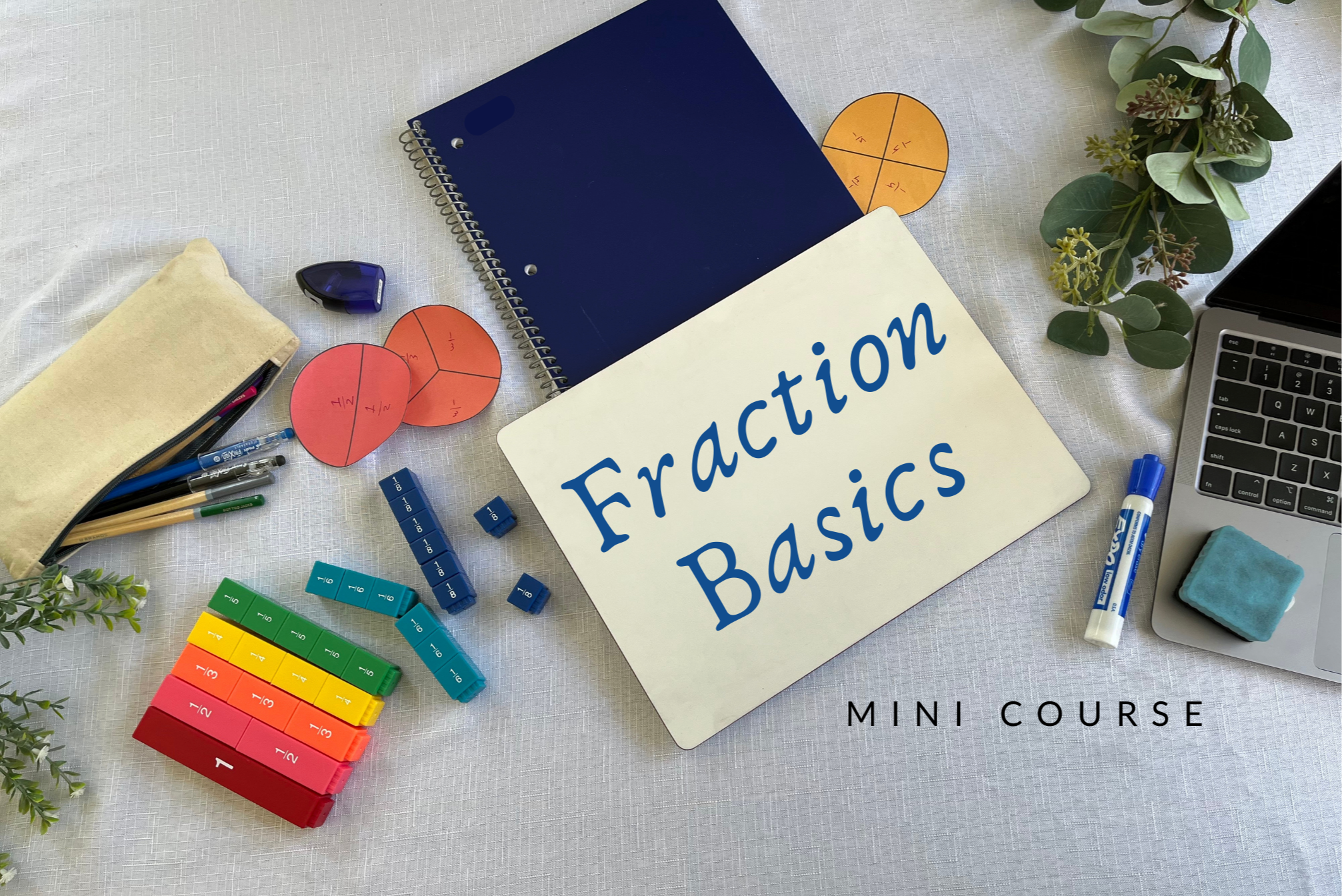## Math Basics

If your child struggles with basic math concepts or needs to practice over the summer, this is the perfect Mini Course for them.

In the Math Basics Mini Course, we will cover place value, multiplication with one and two-digit multipliers, rounding, long division, factors and multiples, finding common denominators as well as equivalent fractions, and much more.## Algebra Prep

If your child struggled with pre-Algebra or Algebra 1, or simply needs a refresher, this is the right Mini Course for them.

Algebra Prep starts with a review of factors, multiples, and exponents.  Then moves on to cover such topics as order of operations, prime factorization, positive and negative numbers, solving for variables, simplifying equations, distributive property, and much more.